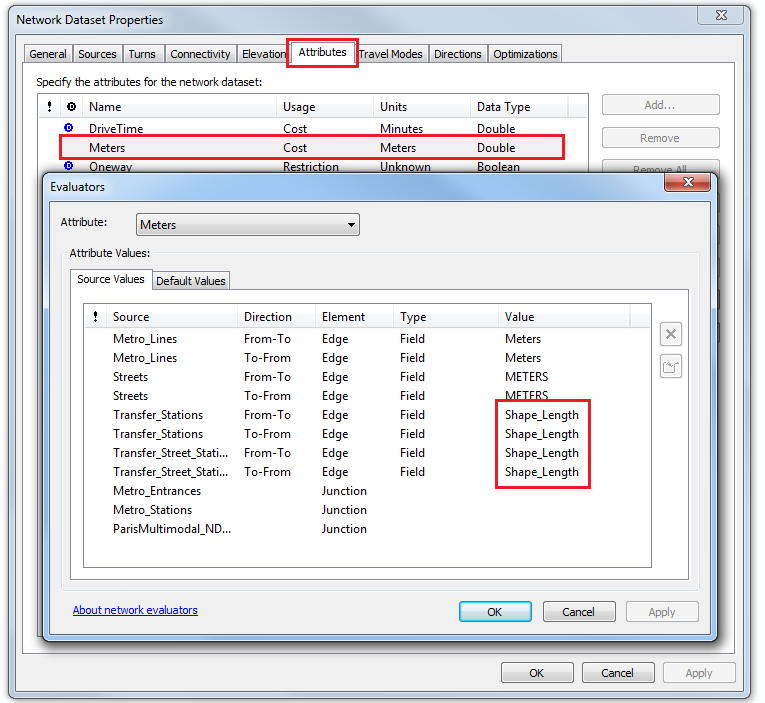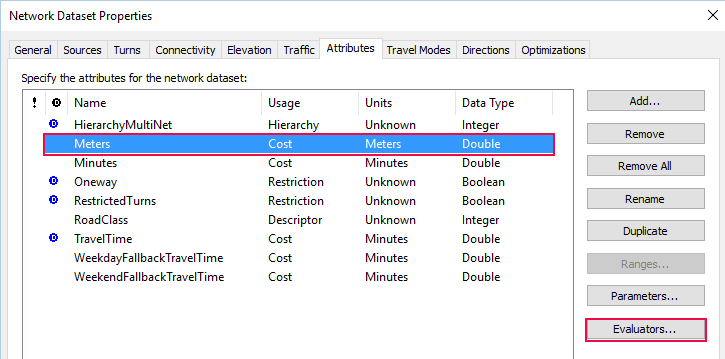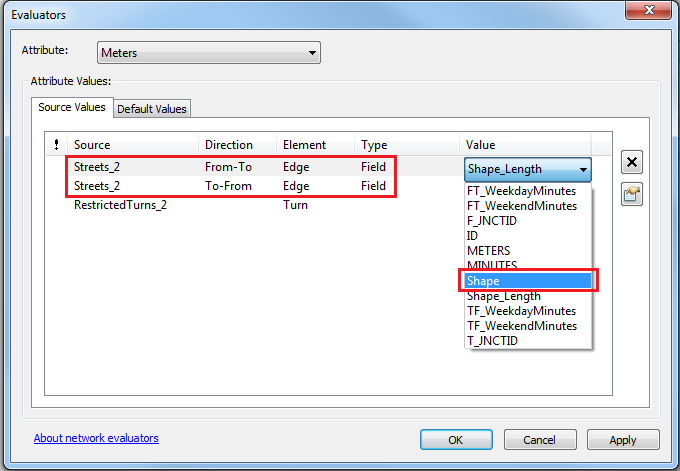English

# Problem: Incorrect distance displayed for directions generated by the Directions tool

## Description

The Directions (Network Analyst) tool generates turn-by-turn directions from a network analysis layer with routes to an output directions file in text, XML, or HTML format. The output directions file includes the distance of each edge in a route. In some instances, generating directions from a network analysis layer in a geographic coordinate system, which does not have a projection, outputs a directions file containing the incorrect distance for edges in the route. For example, the generated file records a travel distance of 1100 feet down the road for one of the road edges in the route, while the road is only 800 feet long.

## Cause

The evaluator value for the distance cost attribute (in the examples below, it is the Meters network attribute) of the network dataset is set to the Shape_Length field. The distance cost network attribute is configured to get the length values of edges in the network based on the evaluator assigned to the attribute.

```Note:
For more information on the network attributes, refer to Understanding network attributes. To view the evaluator value used by the distance cost network attribute in a network dataset, open the Network Dataset Properties dialog box, and click the Attributes tab > select the distance cost > Evaluators.```The figure above displays the evaluators for the Meters network attribute in which the Shape_Length field is used as the cost attribute value. The Meters evaluator calculates the distance cost for edges in the network and determines how long the edge is based on the value assigned. The Value column in the Evaluators dialog box holds information the evaluator uses to calculate the attribute values.

When using the Shape_Length field for the distance impedance, the directions generator is only able to read the actual length values of the selected field. The directions generator reads the length values of the Shape_Length field in decimal degrees and applies a generic conversion to the values. It does not take into consideration the location of the network dataset from the Equator, as it only reads the length values of the Shape_Length field.

## Solution or Workaround

Use the Shape field as the distance cost attribute value for the distance cost network attribute when working with a network analysis layer in a geographic coordinate system. The directions generator is able to read both the length values and the location of the layer on the Earth when using the Shape field as the distance cost attribute value, which results in a correct calculation for the geodesic length while converting the length values.

The following instructions describe how to set the distance cost attribute value for the Meters network attribute to the Shape field:

1. Open the properties of the network dataset from ArcCatalog or the Catalog window in ArcMap by right-clicking the network dataset > Properties.
2. Open the Attributes tab.
3. Click the distance cost (in this case, Meters) row, and click Evaluators....1. In the Evaluators dialog box, click the Source Values tab.
2. Change the evaluator value for the linear source feature classes, which become edge elements in the network dataset, to the Shape field. Ensure the evaluator Type is set to Field, and select Shape in the Value column as the evaluator.3. Click OK to accept the change to the distance cost.
4. Click OK to accept the changes, and close the Network Dataset properties window.
5. Right-click the network dataset again, and select Build to re-build the network.

Re-solve the route and re-run the Directions tool for the route created using the new network dataset to get the correct distance for edges in the route.